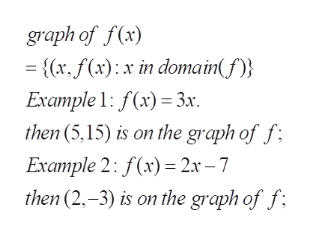# If the point (9,-14) is on the graph of a function f, then f(9)= What is the value of f(9)?

Question

If the point (9,-14) is on the graph of a function f, then f(9)=

What is the value of f(9)?

check_circleExpert Solution
Step 1

To read the values of the function f(x) at a specified point  from its graph

Step 2help_outlineImage Transcriptionclosegraph of f(x) - {x, f(x) x in domain(f Example 1: f(x)3x then(5,15) is on the graph of f Example 2: f(x) 2x -7 then (2,-3) is on the graph of f fullscreen

### Want to see the full answer?

See Solution

#### Want to see this answer and more?

Solutions are written by subject experts who are available 24/7. Questions are typically answered within 1 hour*

See Solution
*Response times may vary by subject and question
Tagged in

### Algebra